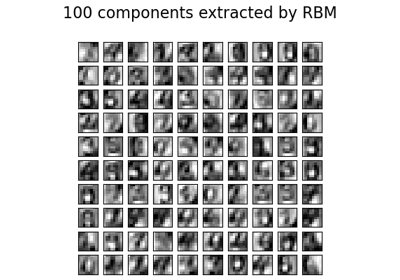# sklearn.neural_network.BernoulliRBM¶

class sklearn.neural_network.BernoulliRBM(n_components=256, *, learning_rate=0.1, batch_size=10, n_iter=10, verbose=0, random_state=None)[source]

Bernoulli Restricted Boltzmann Machine (RBM).

A Restricted Boltzmann Machine with binary visible units and binary hidden units. Parameters are estimated using Stochastic Maximum Likelihood (SML), also known as Persistent Contrastive Divergence (PCD) .

The time complexity of this implementation is O(d ** 2) assuming d ~ n_features ~ n_components.

Read more in the User Guide.

Parameters
n_componentsint, default=256

Number of binary hidden units.

learning_ratefloat, default=0.1

The learning rate for weight updates. It is highly recommended to tune this hyper-parameter. Reasonable values are in the 10**[0., -3.] range.

batch_sizeint, default=10

Number of examples per minibatch.

n_iterint, default=10

Number of iterations/sweeps over the training dataset to perform during training.

verboseint, default=0

The verbosity level. The default, zero, means silent mode.

random_stateinteger or RandomState, default=None

Determines random number generation for:

• Gibbs sampling from visible and hidden layers.

• Initializing components, sampling from layers during fit.

• Corrupting the data when scoring samples.

Pass an int for reproducible results across multiple function calls. See Glossary.

Attributes
intercept_hidden_array-like, shape (n_components,)

Biases of the hidden units.

intercept_visible_array-like, shape (n_features,)

Biases of the visible units.

components_array-like, shape (n_components, n_features)

Weight matrix, where n_features in the number of visible units and n_components is the number of hidden units.

h_samples_array-like, shape (batch_size, n_components)

Hidden Activation sampled from the model distribution, where batch_size in the number of examples per minibatch and n_components is the number of hidden units.

References

 Hinton, G. E., Osindero, S. and Teh, Y. A fast learning algorithm for

deep belief nets. Neural Computation 18, pp 1527-1554. https://www.cs.toronto.edu/~hinton/absps/fastnc.pdf

 Tieleman, T. Training Restricted Boltzmann Machines using

Approximations to the Likelihood Gradient. International Conference on Machine Learning (ICML) 2008

Examples

>>> import numpy as np
>>> from sklearn.neural_network import BernoulliRBM
>>> X = np.array([[0, 0, 0], [0, 1, 1], [1, 0, 1], [1, 1, 1]])
>>> model = BernoulliRBM(n_components=2)
>>> model.fit(X)
BernoulliRBM(n_components=2)


Methods

 fit(X[, y]) Fit the model to the data X. fit_transform(X[, y]) Fit to data, then transform it. get_params([deep]) Get parameters for this estimator. Perform one Gibbs sampling step. partial_fit(X[, y]) Fit the model to the data X which should contain a partial segment of the data. Compute the pseudo-likelihood of X. set_params(**params) Set the parameters of this estimator. Compute the hidden layer activation probabilities, P(h=1|v=X).
__init__(n_components=256, *, learning_rate=0.1, batch_size=10, n_iter=10, verbose=0, random_state=None)[source]

Initialize self. See help(type(self)) for accurate signature.

fit(X, y=None)[source]

Fit the model to the data X.

Parameters
X{array-like, sparse matrix} of shape (n_samples, n_features)

Training data.

Returns
selfBernoulliRBM

The fitted model.

fit_transform(X, y=None, **fit_params)[source]

Fit to data, then transform it.

Fits transformer to X and y with optional parameters fit_params and returns a transformed version of X.

Parameters
X{array-like, sparse matrix, dataframe} of shape (n_samples, n_features)
yndarray of shape (n_samples,), default=None

Target values.

**fit_paramsdict

Returns
X_newndarray array of shape (n_samples, n_features_new)

Transformed array.

get_params(deep=True)[source]

Get parameters for this estimator.

Parameters
deepbool, default=True

If True, will return the parameters for this estimator and contained subobjects that are estimators.

Returns
paramsmapping of string to any

Parameter names mapped to their values.

gibbs(v)[source]

Perform one Gibbs sampling step.

Parameters
vndarray of shape (n_samples, n_features)

Values of the visible layer to start from.

Returns
v_newndarray of shape (n_samples, n_features)

Values of the visible layer after one Gibbs step.

partial_fit(X, y=None)[source]

Fit the model to the data X which should contain a partial segment of the data.

Parameters
Xndarray of shape (n_samples, n_features)

Training data.

Returns
selfBernoulliRBM

The fitted model.

score_samples(X)[source]

Compute the pseudo-likelihood of X.

Parameters
X{array-like, sparse matrix} of shape (n_samples, n_features)

Values of the visible layer. Must be all-boolean (not checked).

Returns
pseudo_likelihoodndarray of shape (n_samples,)

Value of the pseudo-likelihood (proxy for likelihood).

Notes

This method is not deterministic: it computes a quantity called the free energy on X, then on a randomly corrupted version of X, and returns the log of the logistic function of the difference.

set_params(**params)[source]

Set the parameters of this estimator.

The method works on simple estimators as well as on nested objects (such as pipelines). The latter have parameters of the form <component>__<parameter> so that it’s possible to update each component of a nested object.

Parameters
**paramsdict

Estimator parameters.

Returns
selfobject

Estimator instance.

transform(X)[source]

Compute the hidden layer activation probabilities, P(h=1|v=X).

Parameters
X{array-like, sparse matrix} of shape (n_samples, n_features)

The data to be transformed.

Returns
hndarray of shape (n_samples, n_components)

Latent representations of the data.

## Examples using sklearn.neural_network.BernoulliRBM¶